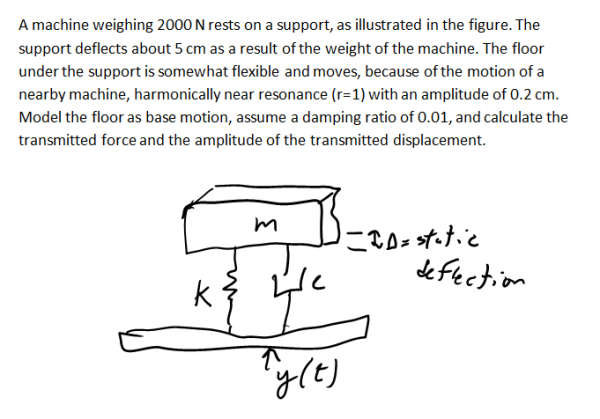A machine weighing 2000 N rests on a support, as illustrated in the fiugre. The support deflects about 5 cm as a result of the weight of the machine. The floor under the support is somewhat flexible and moves, because of the motion of a nearby machine, harmonically near resonance (r=1) with an amplitude of 0.2 cm. Model the floor as base motion, assume a damping ratio of 0.01, and calculate the transmitted force and the amplitude of the transmitted displacement.A machine weighing 2000 N rests on a support, as illustrated in the fiugre. The support deflects about 5 cm as a result of the weight of the machine. The floor under the support is somewhat flexible and moves, because of the motion of a nearby machine, harmonically near resonance (r=1) with an amplitude of 0.2 cm. Model the floor as base motion, assume a damping ratio of 0.01, and calculate the transmitted force and the amplitude of the transmitted displacement.

Vibrations Page 1 vibrations vibrations vibrations vibrations vibrations vibrations vibrations Vibrations Page 2